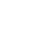# Kotlin Algorithm Challenge #1Alex Woods

December 19, 2019

The following problem is the Add Two Numbers problem on LeetCode.

## Problem

You are given two non-empty linked lists representing two non-negative integers. The digits are stored in reverse order and each of their nodes contain a single digit. Add the two numbers and return it as a linked list.

You may assume the two numbers do not contain any leading zero, except the number 0 itself.

For example:

``````Input: (2 -> 4 -> 3) + (5 -> 6 -> 4)
Output: 7 -> 0 -> 8
Explanation: 342 + 465 = 807.
``````

We’ll use the following class as the basis for our linked list.

``````data class ListNode(val num: Int, val next: ListNode?)

// 342, would be 2 -> 4 -> 3
ListNode(2, ListNode(4, ListNode(3, null)))
``````

## Collapse Function

In order to add the 2 linked lists, we’ll convert them to numbers, then add them, then convert the result back to a linked list.

As a first step, let’s create a function that takes in a ListNode, and returns the integer it represents.

The goal here is to iterate through the list, maintaining a sum of the final number. With each iteration, we add the value of the current node (multiplied by a factor of 10) to the running total. The factor of 10 is just 10 to the power of it’s place in the list.Note that we’re iterating through a linked list, so we’ll do that with a `while` loop until the `node.next = null`.

``````import kotlin.math.pow

fun collapse(node: ListNode): Int {
var runningTotal = 0
var current: ListNode? = node
var i = 0

while (current != null) {
runningTotal += (10.0.pow(i).toInt() * current.num)

i++
current = current.next
}

return runningTotal
}
``````

Let’s check if our function works as imagined. Recall that the numbers in the linked list are the starting at the least significant digits.

``````collapse(ListNode(4, null))                              // 4
collapse(ListNode(4, ListNode(5, null)))                 // 54
collapse(ListNode(4, ListNode(5, ListNode(3, null))))    // 354
``````

## Expand Function

Conversely, let’s write a function that takes in an integer and returns a `ListNode`. This’ll be useful because the final result is supposed to be a `ListNode`. Also it helps us with testing.

As with the `collapse` function, there are two main approaches we could take to go to and from the linked list to an integer.

The easiest data structure to handle this problem is an list of the digits. In order to get there, we could take the numeric route using powers of 10 like we did in the `collapse` function, but it’s simpler to just use string parsing.

Once we have a list of the digits, then we just need to reduce the list to a single list node. For this, we’re going to use the Kotlin `fold` function.

`fold` is very similar to `reduce`, but reduce takes the first value we’re iterating over as the initial value, whereas with `fold` we can specify our own. In our case this is important, because we want the final value to be a `ListNode`, not an `Int`.

So, we’ll create a list node with the first digit and use it as our initial value, e.g. `ListNode(digits.get(0), null)`.

``````fun expand(num: Int): ListNode {
val digits: List<Int> = num.toString().toCharArray().map { it.toString().toInt() }.toList()

return digits
.subList(1, digits.size)
.fold(ListNode(digits.get(0), null)) { acc, current -> ListNode(current, acc) }
}
``````
``````expand(342)   // ListNode(num=2, next=ListNode(num=4, next=ListNode(num=3, next=null)))
expand(76)    // ListNode(num=6, next=ListNode(num=7, next=null))
``````

Notice how clean the output is. This is because we specified the `ListNode` class as a `data` class. This overcomes one of the main daily annoyances of working with Java—classes that have useless `toString()` functions. If we hadn’t specified the class as a `data` class, the output would look like this: `ListNode@48bc2fce`. Which is useless.

Lastly, just for a sanity check, let’s use our `collapse` and `expand` function in conjunction.

``````collapse(expand(76))    // 76
``````

Awesome.

# A Better Solution

Gonna be honest, the first time I did this problem, the above was my solution. But 30 minutes after I published this, it was gnawing at me that I had to go collapse and expand the lists, going through them multiple times.

A few things made me think I was on the wrong path:

• They want you to return a linked list. Why would I go to a number, then re-expand to a linked list?
• There’s really no reason you can’t traverse both at the same time, although you do have to maintain state because the sum of two digits can be greater than 9, meaning we’d carry over to the next digit.

Here’s my final solution; it’s simpler, but a little tougher to follow. Let’s create a recursive helper function, that takes in 2 nodes, and adds them together, and allows us to pass state through in a function parameter.

``````fun addTwoNodes(x: ListNode?, y: ListNode?, carry: Int): ListNode? {
if (x == null && y == null && carry == 0) {
return null
}

val sum = (x?.num ?: 0) + (y?.num ?: 0) + carry

return ListNode(sum % 10, addTwoNodes(x?.next, y?.next, sum / 10))
}
``````

This function is much cleaner—there's no while loop. The key things that might be confusing are Kotlin’s null safety and the elvis operator.

Anyway, once we have this helper function, we get a `O(log_10(x + y))` solution.

``````fun addTwoNumbers(l1: ListNode?, l2: ListNode?): ListNode {